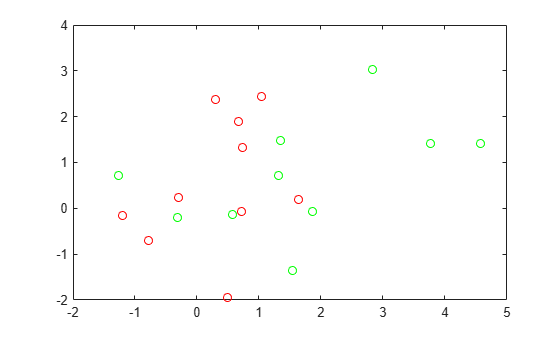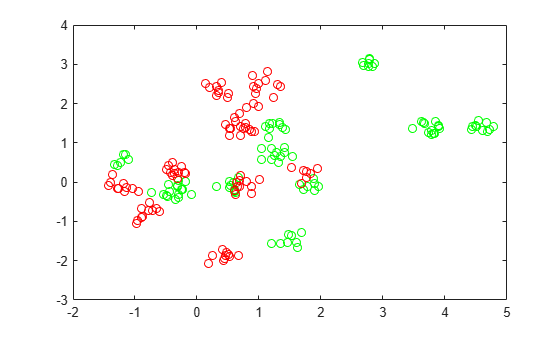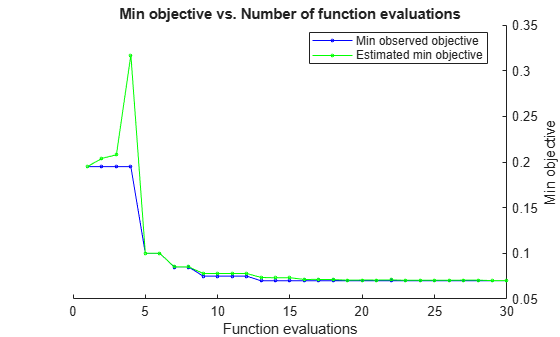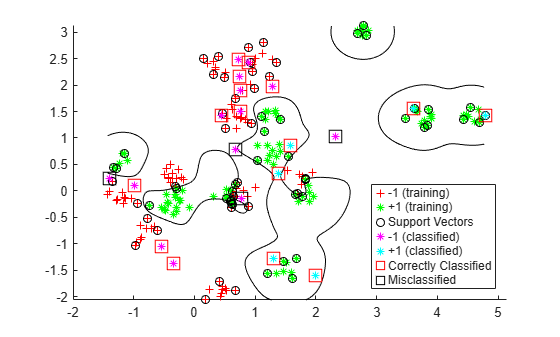# Optimize Classifier Fit Using Bayesian Optimization

This example shows how to optimize an SVM classification using the `fitcsvm` function and the `OptimizeHyperparameters` name-value argument.

### Generate Data

The classification works on locations of points from a Gaussian mixture model. In The Elements of Statistical Learning, Hastie, Tibshirani, and Friedman (2009), page 17 describes the model. The model begins with generating 10 base points for a "green" class, distributed as 2-D independent normals with mean (1,0) and unit variance. It also generates 10 base points for a "red" class, distributed as 2-D independent normals with mean (0,1) and unit variance. For each class (green and red), generate 100 random points as follows:

1. Choose a base point m of the appropriate color uniformly at random.

2. Generate an independent random point with 2-D normal distribution with mean m and variance I/5, where I is the 2-by-2 identity matrix. In this example, use a variance I/50 to show the advantage of optimization more clearly.

Generate the 10 base points for each class.

```rng('default') % For reproducibility grnpop = mvnrnd([1,0],eye(2),10); redpop = mvnrnd([0,1],eye(2),10);```

View the base points.

```plot(grnpop(:,1),grnpop(:,2),'go') hold on plot(redpop(:,1),redpop(:,2),'ro') hold off```Since some red base points are close to green base points, it can be difficult to classify the data points based on location alone.

Generate the 100 data points of each class.

```redpts = zeros(100,2); grnpts = redpts; for i = 1:100 grnpts(i,:) = mvnrnd(grnpop(randi(10),:),eye(2)*0.02); redpts(i,:) = mvnrnd(redpop(randi(10),:),eye(2)*0.02); end```

View the data points.

```figure plot(grnpts(:,1),grnpts(:,2),'go') hold on plot(redpts(:,1),redpts(:,2),'ro') hold off```### Prepare Data for Classification

Put the data into one matrix, and make a vector `grp` that labels the class of each point. 1 indicates the green class, and –1 indicates the red class.

```cdata = [grnpts;redpts]; grp = ones(200,1); grp(101:200) = -1;```

### Prepare Cross-Validation

Set up a partition for cross-validation.

`c = cvpartition(200,'KFold',10);`

This step is optional. If you specify a partition for the optimization, then you can compute an actual cross-validation loss for the returned model.

### Optimize Fit

To find a good fit, meaning one with optimal hyperparameters that minimize the cross-validation loss, use Bayesian optimization. Specify a list of hyperparameters to optimize by using the `OptimizeHyperparameters` name-value argument, and specify optimization options by using the `HyperparameterOptimizationOptions` name-value argument.

Specify `'OptimizeHyperparameters'` as `'auto'`. The `'auto'` option includes a typical set of hyperparameters to optimize. `fitcsvm` finds optimal values of `BoxConstraint` and `KernelScale`. Set the hyperparameter optimization options to use the cross-validation partition `c` and to choose the `'expected-improvement-plus'` acquisition function for reproducibility. The default acquisition function depends on run time and, therefore, can give varying results.

```opts = struct('CVPartition',c,'AcquisitionFunctionName','expected-improvement-plus'); Mdl = fitcsvm(cdata,grp,'KernelFunction','rbf', ... 'OptimizeHyperparameters','auto','HyperparameterOptimizationOptions',opts)```
```|=====================================================================================================| | Iter | Eval | Objective | Objective | BestSoFar | BestSoFar | BoxConstraint| KernelScale | | | result | | runtime | (observed) | (estim.) | | | |=====================================================================================================| | 1 | Best | 0.345 | 0.15259 | 0.345 | 0.345 | 0.00474 | 306.44 | | 2 | Best | 0.115 | 0.10129 | 0.115 | 0.12678 | 430.31 | 1.4864 | | 3 | Accept | 0.52 | 0.19601 | 0.115 | 0.1152 | 0.028415 | 0.014369 | | 4 | Accept | 0.61 | 0.19179 | 0.115 | 0.11504 | 133.94 | 0.0031427 | | 5 | Accept | 0.34 | 0.2641 | 0.115 | 0.11504 | 0.010993 | 5.7742 | | 6 | Best | 0.085 | 0.13069 | 0.085 | 0.085039 | 885.63 | 0.68403 | | 7 | Accept | 0.105 | 0.16678 | 0.085 | 0.085428 | 0.3057 | 0.58118 | | 8 | Accept | 0.21 | 0.19151 | 0.085 | 0.09566 | 0.16044 | 0.91824 | | 9 | Accept | 0.085 | 0.19728 | 0.085 | 0.08725 | 972.19 | 0.46259 | | 10 | Accept | 0.1 | 0.24964 | 0.085 | 0.090952 | 990.29 | 0.491 | | 11 | Best | 0.08 | 0.17037 | 0.08 | 0.079362 | 2.5195 | 0.291 | | 12 | Accept | 0.09 | 0.12204 | 0.08 | 0.08402 | 14.338 | 0.44386 | | 13 | Accept | 0.1 | 0.16216 | 0.08 | 0.08508 | 0.0022577 | 0.23803 | | 14 | Accept | 0.11 | 0.23621 | 0.08 | 0.087378 | 0.2115 | 0.32109 | | 15 | Best | 0.07 | 0.18117 | 0.07 | 0.081507 | 910.2 | 0.25218 | | 16 | Best | 0.065 | 0.18389 | 0.065 | 0.072457 | 953.22 | 0.26253 | | 17 | Accept | 0.075 | 0.27043 | 0.065 | 0.072554 | 998.74 | 0.23087 | | 18 | Accept | 0.295 | 0.17016 | 0.065 | 0.072647 | 996.18 | 44.626 | | 19 | Accept | 0.07 | 0.17971 | 0.065 | 0.06946 | 985.37 | 0.27389 | | 20 | Accept | 0.165 | 0.20585 | 0.065 | 0.071622 | 0.065103 | 0.13679 | |=====================================================================================================| | Iter | Eval | Objective | Objective | BestSoFar | BestSoFar | BoxConstraint| KernelScale | | | result | | runtime | (observed) | (estim.) | | | |=====================================================================================================| | 21 | Accept | 0.345 | 0.16283 | 0.065 | 0.071764 | 971.7 | 999.01 | | 22 | Accept | 0.61 | 0.18862 | 0.065 | 0.071967 | 0.0010168 | 0.0010005 | | 23 | Accept | 0.345 | 0.17211 | 0.065 | 0.071959 | 0.0011459 | 995.89 | | 24 | Accept | 0.35 | 0.1867 | 0.065 | 0.071863 | 0.0010003 | 40.628 | | 25 | Accept | 0.24 | 0.31191 | 0.065 | 0.072124 | 996.55 | 10.423 | | 26 | Accept | 0.61 | 0.1737 | 0.065 | 0.072067 | 994.71 | 0.0010063 | | 27 | Accept | 0.47 | 0.12519 | 0.065 | 0.07218 | 993.69 | 0.029723 | | 28 | Accept | 0.3 | 0.12101 | 0.065 | 0.072291 | 993.15 | 170.01 | | 29 | Accept | 0.16 | 0.26368 | 0.065 | 0.072103 | 992.81 | 3.8594 | | 30 | Accept | 0.365 | 0.18218 | 0.065 | 0.072112 | 0.0010017 | 0.044287 | ``````__________________________________________________________ Optimization completed. MaxObjectiveEvaluations of 30 reached. Total function evaluations: 30 Total elapsed time: 27.3487 seconds Total objective function evaluation time: 5.6116 Best observed feasible point: BoxConstraint KernelScale _____________ ___________ 953.22 0.26253 Observed objective function value = 0.065 Estimated objective function value = 0.073726 Function evaluation time = 0.18389 Best estimated feasible point (according to models): BoxConstraint KernelScale _____________ ___________ 985.37 0.27389 Estimated objective function value = 0.072112 Estimated function evaluation time = 0.18119 ```
```Mdl = ClassificationSVM ResponseName: 'Y' CategoricalPredictors: [] ClassNames: [-1 1] ScoreTransform: 'none' NumObservations: 200 HyperparameterOptimizationResults: [1x1 BayesianOptimization] Alpha: [77x1 double] Bias: -0.2352 KernelParameters: [1x1 struct] BoxConstraints: [200x1 double] ConvergenceInfo: [1x1 struct] IsSupportVector: [200x1 logical] Solver: 'SMO' Properties, Methods ```

`fitcsvm` returns a `ClassificationSVM` model object that uses the best estimated feasible point. The best estimated feasible point is the set of hyperparameters that minimizes the upper confidence bound of the cross-validation loss based on the underlying Gaussian process model of the Bayesian optimization process.

The Bayesian optimization process internally maintains a Gaussian process model of the objective function. The objective function is the cross-validated misclassification rate for classification. For each iteration, the optimization process updates the Gaussian process model and uses the model to find a new set of hyperparameters. Each line of the iterative display shows the new set of hyperparameters and these column values:

• `Objective` — Objective function value computed at the new set of hyperparameters.

• `Objective runtime` — Objective function evaluation time.

• `Eval result` — Result report, specified as `Accept`, `Best`, or `Error`. `Accept` indicates that the objective function returns a finite value, and `Error` indicates that the objective function returns a value that is not a finite real scalar. `Best` indicates that the objective function returns a finite value that is lower than previously computed objective function values.

• `BestSoFar(observed)` — The minimum objective function value computed so far. This value is either the objective function value of the current iteration (if the `Eval result` value for the current iteration is `Best`) or the value of the previous `Best` iteration.

• `BestSoFar(estim.)` — At each iteration, the software estimates the upper confidence bounds of the objective function values, using the updated Gaussian process model, at all the sets of hyperparameters tried so far. Then the software chooses the point with the minimum upper confidence bound. The `BestSoFar(estim.)` value is the objective function value returned by the `predictObjective` function at the minimum point.

The plot below the iterative display shows the `BestSoFar(observed)` and `BestSoFar(estim.)` values in blue and green, respectively.

The returned object `Mdl` uses the best estimated feasible point, that is, the set of hyperparameters that produces the `BestSoFar(estim.)` value in the final iteration based on the final Gaussian process model.

You can obtain the best point from the `HyperparameterOptimizationResults` property or by using the `bestPoint` function.

`Mdl.HyperparameterOptimizationResults.XAtMinEstimatedObjective`
```ans=1×2 table BoxConstraint KernelScale _____________ ___________ 985.37 0.27389 ```
`[x,CriterionValue,iteration] = bestPoint(Mdl.HyperparameterOptimizationResults)`
```x=1×2 table BoxConstraint KernelScale _____________ ___________ 985.37 0.27389 ```
```CriterionValue = 0.0888 ```
```iteration = 19 ```

By default, the `bestPoint` function uses the `'min-visited-upper-confidence-interval'` criterion. This criterion chooses the hyperparameters obtained from the 19th iteration as the best point. `CriterionValue` is the upper bound of the cross-validated loss computed by the final Gaussian process model. Compute the actual cross-validated loss by using the partition `c`.

```L_MinEstimated = kfoldLoss(fitcsvm(cdata,grp,'CVPartition',c,'KernelFunction','rbf', ... 'BoxConstraint',x.BoxConstraint,'KernelScale',x.KernelScale))```
```L_MinEstimated = 0.0700 ```

The actual cross-validated loss is close to the estimated value. The `Estimated objective function value` is displayed below the plots of the optimization results.

You can also extract the best observed feasible point (that is, the last `Best` point in the iterative display) from the `HyperparameterOptimizationResults` property or by specifying `Criterion` as `'min-observed'`.

`Mdl.HyperparameterOptimizationResults.XAtMinObjective`
```ans=1×2 table BoxConstraint KernelScale _____________ ___________ 953.22 0.26253 ```
`[x_observed,CriterionValue_observed,iteration_observed] = bestPoint(Mdl.HyperparameterOptimizationResults,'Criterion','min-observed')`
```x_observed=1×2 table BoxConstraint KernelScale _____________ ___________ 953.22 0.26253 ```
```CriterionValue_observed = 0.0650 ```
```iteration_observed = 16 ```

The `'min-observed'` criterion chooses the hyperparameters obtained from the 16th iteration as the best point. `CriterionValue_observed` is the actual cross-validated loss computed using the selected hyperparameters. For more information, see the Criterion name-value argument of `bestPoint`.

Visualize the optimized classifier.

```d = 0.02; [x1Grid,x2Grid] = meshgrid(min(cdata(:,1)):d:max(cdata(:,1)), ... min(cdata(:,2)):d:max(cdata(:,2))); xGrid = [x1Grid(:),x2Grid(:)]; [~,scores] = predict(Mdl,xGrid); figure h(1:2) = gscatter(cdata(:,1),cdata(:,2),grp,'rg','+*'); hold on h(3) = plot(cdata(Mdl.IsSupportVector,1), ... cdata(Mdl.IsSupportVector,2),'ko'); contour(x1Grid,x2Grid,reshape(scores(:,2),size(x1Grid)),[0 0],'k'); legend(h,{'-1','+1','Support Vectors'},'Location','Southeast');```### Evaluate Accuracy on New Data

Generate and classify new test data points.

```grnobj = gmdistribution(grnpop,.2*eye(2)); redobj = gmdistribution(redpop,.2*eye(2)); newData = random(grnobj,10); newData = [newData;random(redobj,10)]; grpData = ones(20,1); % green = 1 grpData(11:20) = -1; % red = -1 v = predict(Mdl,newData);```

Compute the misclassification rates on the test data set.

`L_Test = loss(Mdl,newData,grpData)`
```L_Test = 0.3500 ```

Determine which new data points are classified correctly. Format the correctly classified points in red squares and the incorrectly classified points in black squares.

```h(4:5) = gscatter(newData(:,1),newData(:,2),v,'mc','**'); mydiff = (v == grpData); % Classified correctly for ii = mydiff % Plot red squares around correct pts h(6) = plot(newData(ii,1),newData(ii,2),'rs','MarkerSize',12); end for ii = not(mydiff) % Plot black squares around incorrect pts h(7) = plot(newData(ii,1),newData(ii,2),'ks','MarkerSize',12); end legend(h,{'-1 (training)','+1 (training)','Support Vectors', ... '-1 (classified)','+1 (classified)', ... 'Correctly Classified','Misclassified'}, ... 'Location','Southeast'); hold off```